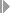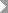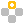Show TOC

###Variances with a Valuated Sales Order StockIf you are using a valuated sales order stock, you can use the functions for variance calculation and the calculation of scrap variances in sales-order-related production.

You can do this in the following situations:

• In mass production on the basis of sales orders with a valuated sales order stock

You do not flag the sales document item as carrying costs and revenues.

In this case the functions of Cost Object Controlling are performed at the product level using manufacturing orders (production orders or process orders) or in repetitive manufacturing using a Product Cost Collectors in Sales-Order-Related Production.

You can also use a cost object hierarchy.

• Complex make-to-order production

Here your costs are managed on a sales document item that carries costs and revenue. That is, you use the Product Cost by Sales Order functionality.

You can calculate variances and unplanned scrap (scrap variances) at the level of the orders (manufacturing orders or product cost collectors) assigned to the sales document item. It is not possible to calculate variances at the level of the sales document item.

#### Prerequisites

If you want to see the Variance Categories rather than just remaining variances, the cost estimate on which the calculation of target costs is based must have an itemization.

This section contains detailed information on the strategy sequence for the selection of the cost estimate relevant to inventory valuation with a valuated sales order stock.

Inventory Valuation on Basis of Sales Order Cost Estimate/Order BOM Cost Estimate

If you want to calculate variances using the standard price for the material manufactured for the sales order that was calculated in a Sales Order Costing or Order BOM Cost Estimate, make sure that:

• The sales order cost estimate / order BOM cost estimate has the status VO (marked)Note

You specify that the status VO (marked) is set automatically in Customizing for Product Cost Controlling underCost Object ControllingProduct Cost by Sales OrderControl of Sales-Order-Related ProductionCheck Requirements Classesby entering B in the Costing ID indicator. This is particularly recommended in mass production environments on the basis of sales orders, because it would involve too much effort to set the status manually.

End of the note.

To be able to calculate scrap variances using a sales order cost estimate, the following requirements must be met:

• This sales order cost estimate/order BOM cost estimate must have been performed with the costing method product costing.

• An itemization must have been updated for this cost estimate.

Inventory Valuation on Basis of Standard Cost Estimate for Collective Requirements Material

If you want the scrap variances to be calculated using a standard price for the material for the sales order which was calculated in a standard cost estimate for the material because you also carry the same material in collective requirements, variance calculation can be performed if the standard cost estimate is marked and released and is valid at the time of variance calculation.

You can calculate variances on the basis of the standard price in the material master even if the standard price was calculated in a material cost estimate without quantity structure. A cost estimate without quantity structure is created with the costing method unit costing. The variances are shown broken down into variance categories.

You can only calculate scrap variances if the standard cost estimate for the material is a material cost estimate with quantity structure (costing method product costing) and it has an itemization.Note

The system requires an itemization with operation information in order to valuate work in process at target costs and scrap variances.

To calculate the total variance, the system only requires a standard price that was calculated in a cost estimate with itemization. If no itemization exists, variance calculation will report all variances as remaining variances. This will be the case for example if the sales order cost estimate is a product cost estimate without an itemization.

End of the note.

#### Features

If you are using a valuated sales order stock, you can calculate the variances the same way as in make-to-stock production:

If you are using a valuated sales order stock, variance calculation calculates the total variances (standard system: target cost version 0) on the basis of the cost estimate that is used to valuate the materials in the sales order stock. This can be the following:

• The standard cost estimate for the material

• A cost estimate selected according to a predefined strategy sequence In the case of the strategy sequence, the system accesses the following cost estimates in the defined sequence.

In variance calculation, the system assumes that the goods receipt is valuated with the standard price. Differences that are due to a different valuation are shown as output price variances. Output price variances are also reported in cases such as the following:Example

You create a sales order cost estimate for a sales order item and mark it. The material is manufactured and placed into finished goods inventory. The standard price is updated.

You then reverse the goods receipt. You create a new sales order cost estimate and mark it. However, you overlooked the fact that once the standard price for the sales order stock is updated, it can no longer be changed automatically. The material is again placed into finished goods inventory. The inventory is valuated with the standard price that was updated with the first goods receipt.

In the period-end closing process of Cost Object Controlling, you calculate the variances. The difference between the standard price and the second sales order cost estimate is reported as an output price variance.

End of the example.

Manual changes to the price of a make-to-order material have the following effects:Example

We continue with the above example:

Suppose you would like to prevent the output price variance from being reported. You manually adjust the standard price of the make-to-order material to the value of the second sales order cost estimate.

Assume that the sales order cost estimate has a total value of EUR 50. The fixed costs are EUR 10 and the variable costs are EUR 40.

When it calculates the variances, the system reports a variance of zero for the variance category output price variance. However, it does report a variance of EUR 10 for the fixed costs and the same amount for the variable costs; these amounts cancel each other out.

End of the example.
##### Constraints

If you are using product cost collectors in sales-order-related production, you can only calculate variances with target cost version 0 if you have created a standard cost estimate for the configurable material.

You cannot calculate variances for the sales order item.Note

If the sales order item carries costs and revenues, the price differences for externally procured individual requirements materials are posted to the sales order item (and not directly to CO-PA). If you now want to see the total actual cost on the sales order item, you must also settle the total variances on the manufacturing orders or product cost collector (the value that is posted to the price difference account) to the sales order item. In this case, make sure you do not settle the total variances to CO-PA because the variances would then get transferred into CO-PA twice.

For more information, read the following section: Settlement in Product Cost by Order or Period

End of the note.